# IB DP Physics: HL复习笔记7.1.2 Calculating Discrete Energies

### Transitions Between Energy Levels

#### Difference in discrete energy levels

• The difference between two energy levels is equal to a specific photon energy
• The energy (hf) of the photon is given by:

ΔE = hf = E2 - E1

• Where:
• E1 = Energy of the higher level (J)
• E2 = Energy of the lower level (J)
• h = Planck's constant (J s)
• f = Frequency of photon (Hz)
• Using the wave equation, the wavelength of the emitted, or absorbed, radiation can be related to the energy difference by the equation:• This equation shows that the larger the difference in energy of two levels ΔE (E2 - E1) the shorter the wavelength λ and vice versa

#### Worked Example

Some electron energy levels in atomic hydrogen are shown below.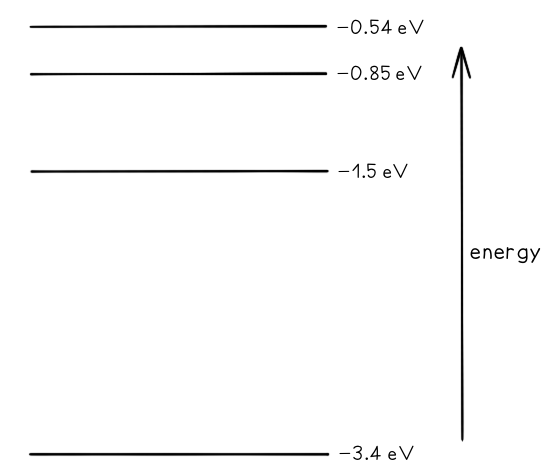The longest wavelength produced as a result of electron transitions between two of the energy levels is 4.0 × 10–6 m.

a) Draw and mark:

• The transition giving rise to the wavelength of 4.0 × 10–6 m with letter L.
• The transition giving rise to the shortest wavelength with letter S.

b) Calculate the wavelength for the transition giving rise to the shortest wavelength.

Part (a)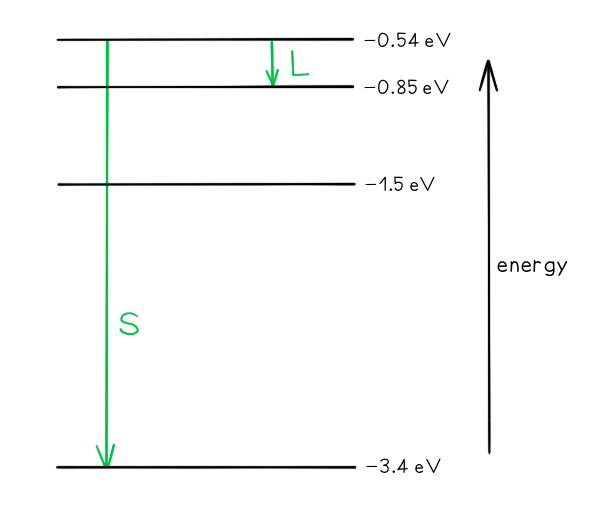• Photon energy and wavelength are inversely proportional
• Therefore, the largest energy change corresponds to the shortest wavelength (line S)
• The smallest energy change corresponds to the longest wavelength (line L)

Part (b)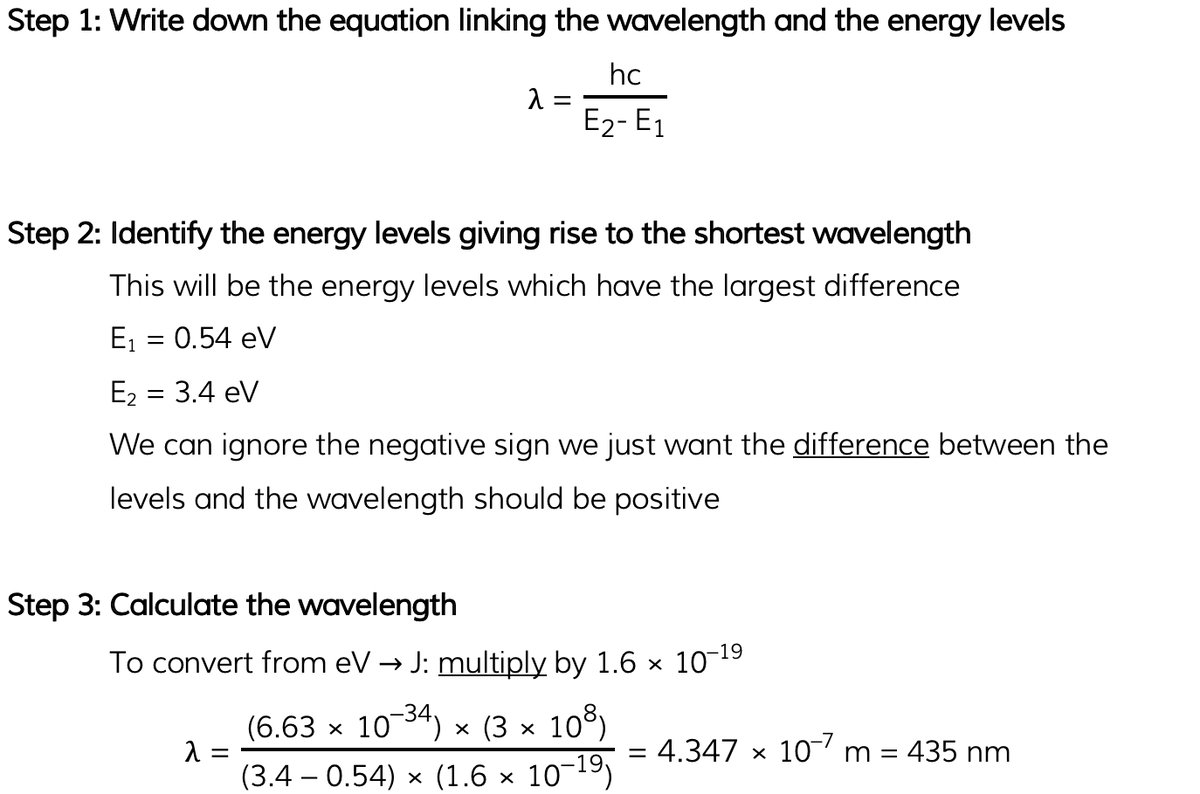#### Worked Example

Given the following electron energy levels in an atom shown below, what is the number of possible wavelengths that can be produced from an electron moving between the fourth excited state and the ground state?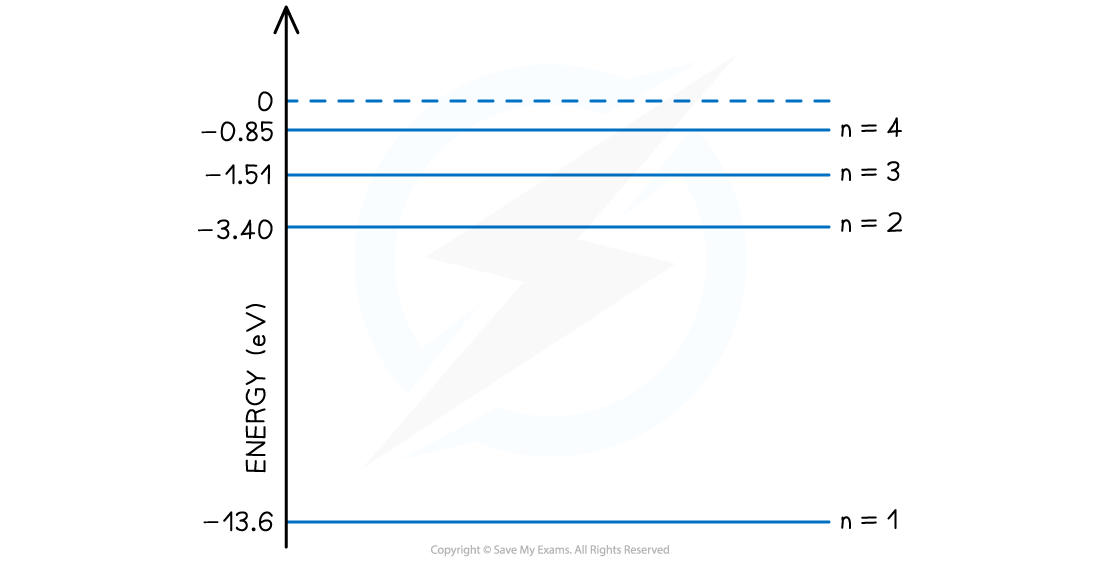• Consider the different ways that the electron may become less excited and emit photons

Step 1: Consider first the case where the electron goes directly from the fourth energy level (n = 4) to the ground state (n = 1)

• This is the first way that the electron may move between these energy levels
• Therefore, this is the highest energy photon that could be emitted since it has the highest energy change
• Each arrow downwards represents a different wavelength of photon released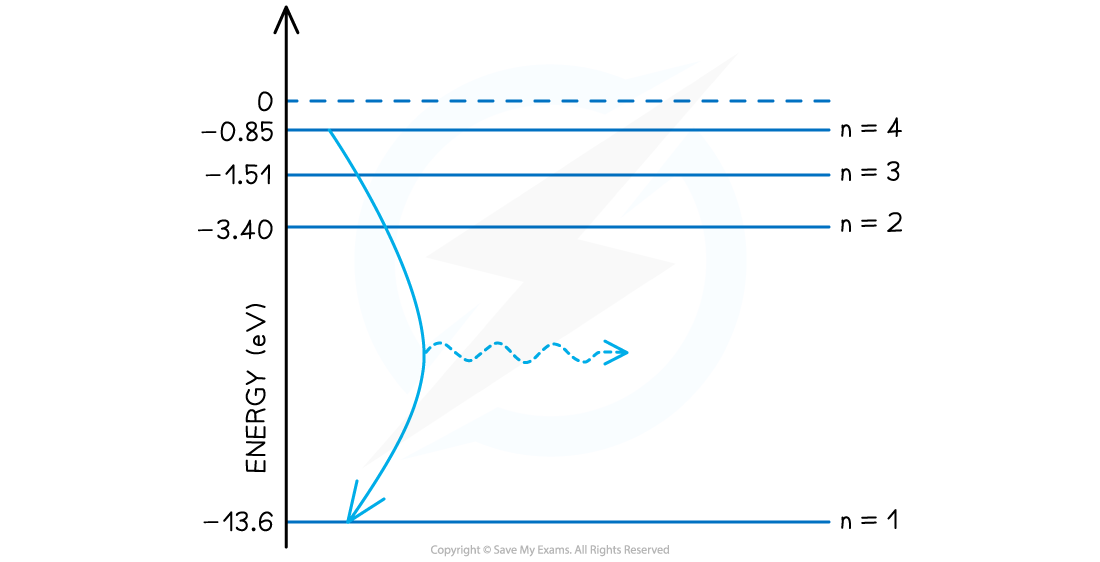Step 2: Consider the cases where two energy level changes are used

• The first is from the fourth energy level (n = 4) to the third energy level (n = 3) and then on to the ground state (n = 1)
• The second is from the fourth energy level (n = 4) to the second energy level (n = 2) and then on to the ground state (n = 1)
• These pathways produce four different photons of different wavelengths and energy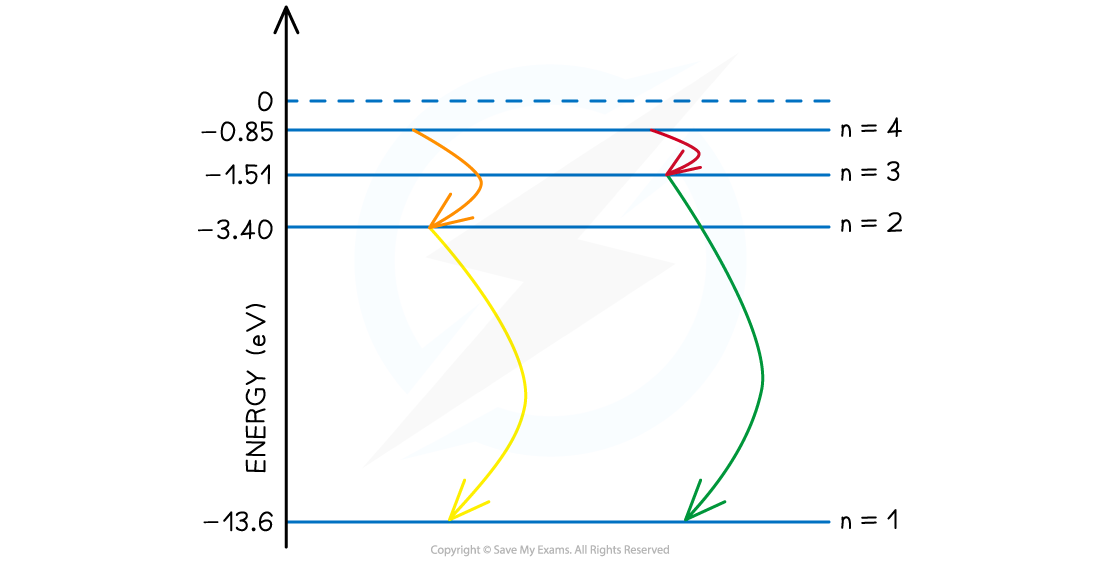Step 3: Consider the case where all possible energy level changes are used

• In this final case, the electron could move down through every energy level, the fourth level to third, then second and ground state. (n = 4 to 3 to 2 to 1).
• While the energies of the photons from n = 4 to 3 and n = 2 to 1 are already accounted for in the previous step, one new photon of a different wavelength is produced in the transition from n = 3 to 2.Step 4: State the final answer

• There are six possible wavelengths that come from the different energy level transitions